## Question 147 Chapter 4 of +2-B – T.S. Grewal 12 ClassQuestion 147 Chapter 4 of +2-B Miscellaneous 147. From the following informations, calculate Return on Investment or Return on Capital Employed :   Rs Share Capital 5,00,000 Reserves and Surplus 2,50,000 Net Fixed Assets 22,50,000 Non-current Trade Investments 2,50,000 Current Read More …

## Question 146 Chapter 4 of +2-B – T.S. Grewal 12 Class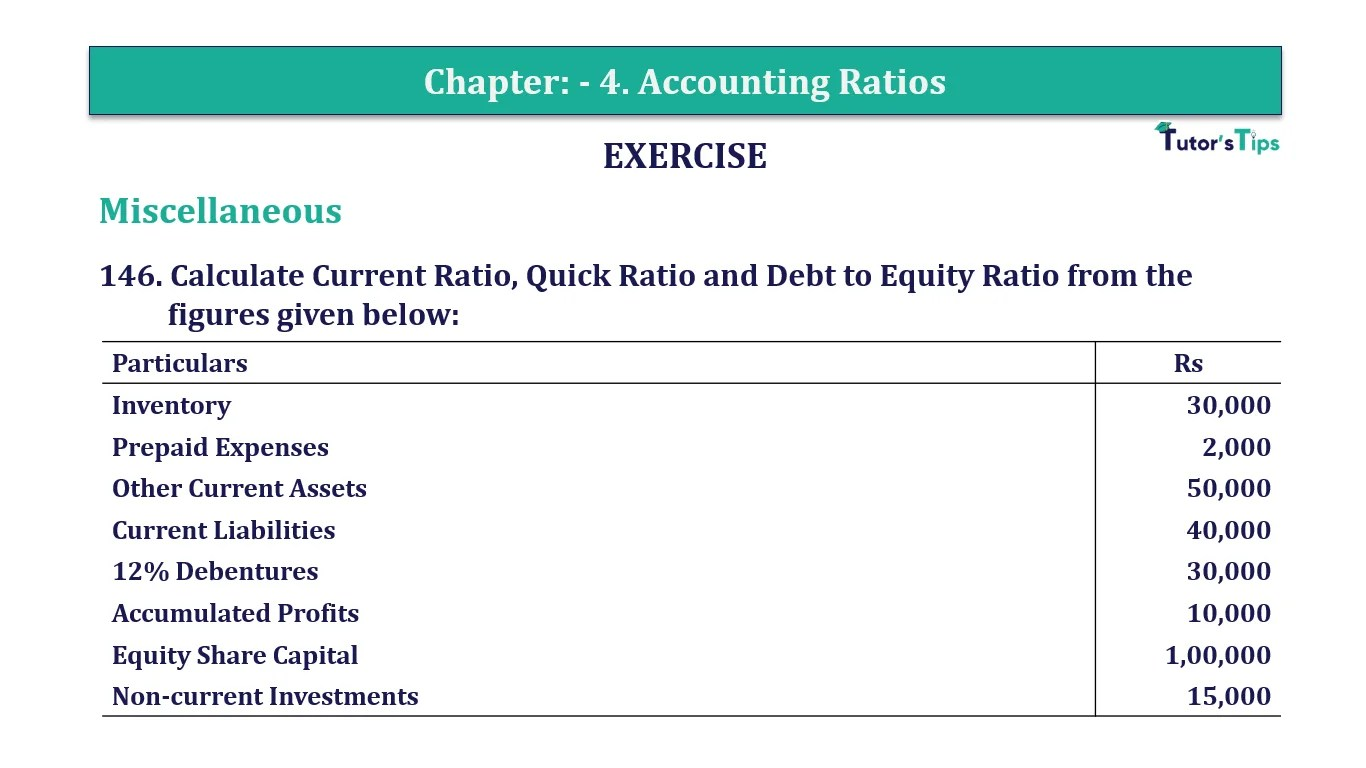Question 146 Chapter 4 of +2-B Miscellaneous 146. Calculate Current Ratio, Quick Ratio and Debt to Equity Ratio from thefigures given below: Particulars Rs Inventory  30,000 Prepaid Expenses 2,000 Other Current Assets 50,000 Current Liabilities 40,000 12% Debentures 30,000 Accumulated Read More …

## Question 145 Chapter 4 of +2-B – T.S. Grewal 12 ClassQuestion 145 Chapter 4 of +2-B Miscellaneous 145. From the following information related to Naveen Ltd., calculate (a) Return on Investment and (b) Total Assets to Debt Ratio:Information: Fixed Assets Rs 75,00,000; Current Assets Rs 40,00,000; Current Liabilities Rs 27,00,000; Read More …

## Question 144 Chapter 4 of +2-B – T.S. Grewal 12 Class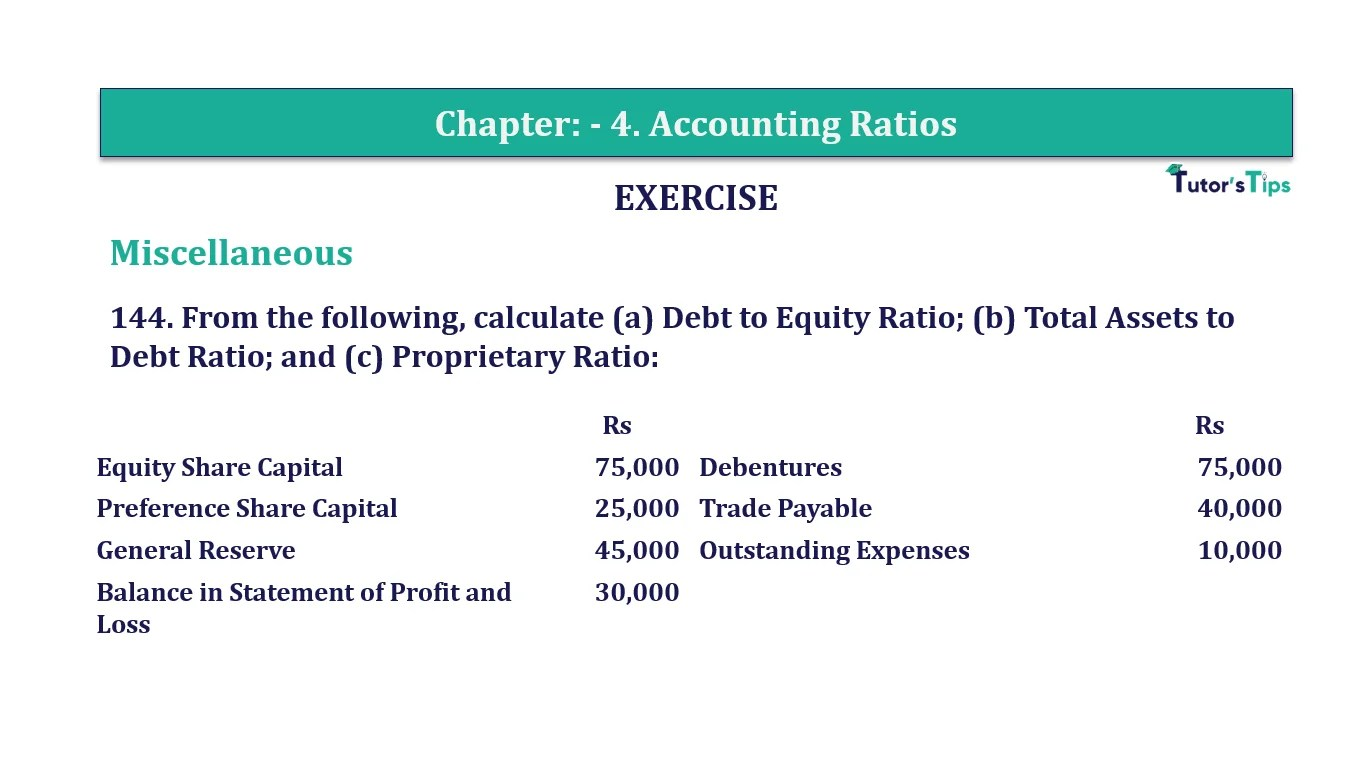Question 144 Chapter 4 of +2-B Miscellaneous 144. From the following, calculate (a) Debt to Equity Ratio; (b) Total Assets to Debt Ratio; and (c) Proprietary Ratio:   Rs   Rs Equity Share Capital  75,000 Debentures 75,000 Preference Share Capital Read More …

## Question 143 Chapter 4 of +2-B – T.S. Grewal 12 ClassQuestion 143 Chapter 4 of +2-B Miscellaneous 143. On the basis of the following information calculate:(i) Debt to Equity Ratio; and(ii) Working Capital Turnover Ratio.Information:     Rs   Rs  Revenue from Operations (a) Cash Sales 40,00,000 Paid-up Share Capital Read More …

## Question 142 Chapter 4 of +2-B – T.S. Grewal 12 Class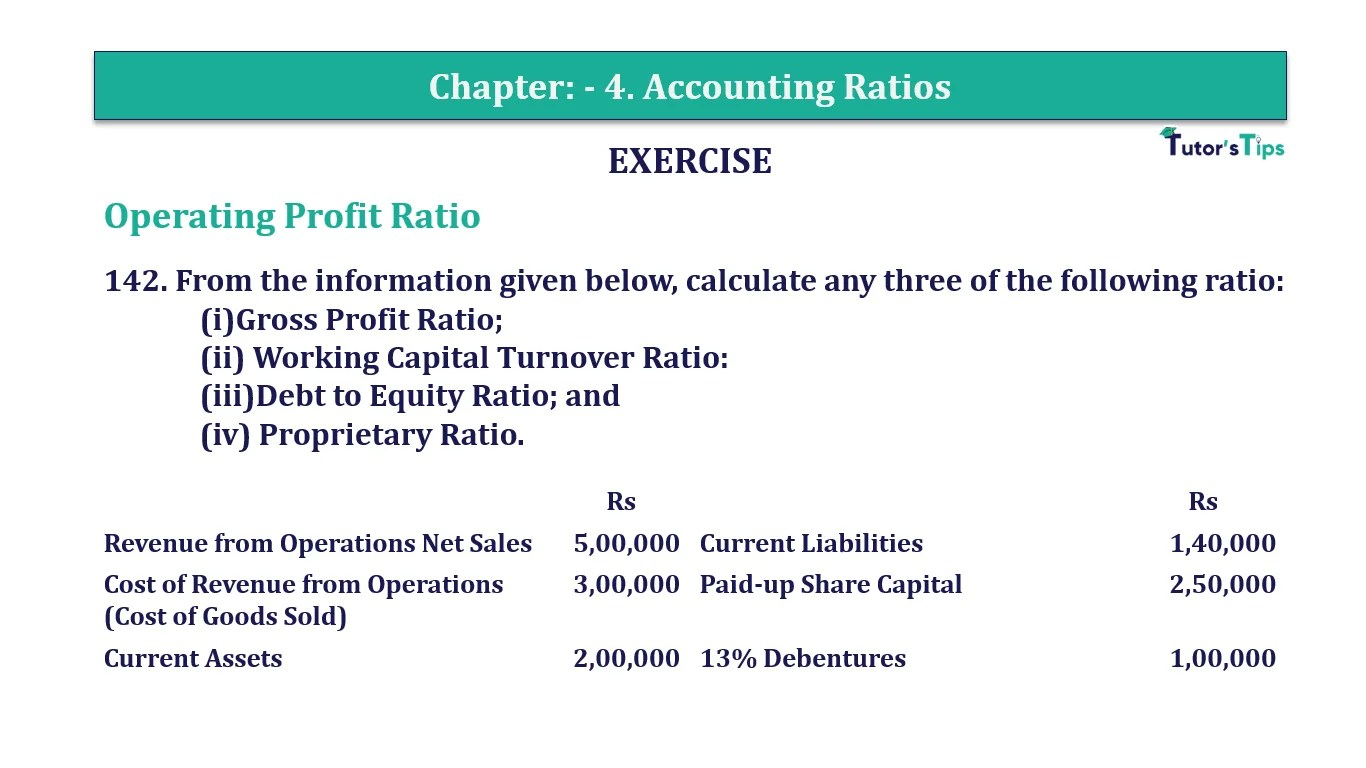Question 142 Chapter 4 of +2-B Miscellaneous 142. From the information given below, calculate any three of the following ratio:(i)Gross Profit Ratio;(ii) Working Capital Turnover Ratio:(iii)Debt to Equity Ratio; and(iv) Proprietary Ratio.   Rs    Rs  Revenue from Operations Net Read More …

## Question 141 Chapter 4 of +2-B – T.S. Grewal 12 ClassQuestion 141 Chapter 4 of +2-B Miscellaneous 141Calculate following ratios on the basis of the given information:(i)Current Ratio;(ii)Acid Test Ratio;(iii)Operating Ratio; and(iv)Gross Profit Ratio.   Rs    Rs Current Assets 70,000 Revenue from Operations Sales 1,20,000 Current Liabilities 35,000 Operating Read More …

## Question 140 Chapter 4 of +2-B – T.S. Grewal 12 ClassQuestion 140 Chapter 4 of +2-B Miscellaneous 140. Calculate following ratios on the basis of the following information:(i)Gross Profit Ratio;(ii)Current Ratio;(iii)Acid Test Ratio; and(iv) Inventory Turnover Ratio.   Rs   Rs Gross Profit 50,000 Revenue from Operations 1,00,000 Inventory 15,000 Read More …

## Question 139 Chapter 4 of +2-B – T.S. Grewal 12 Class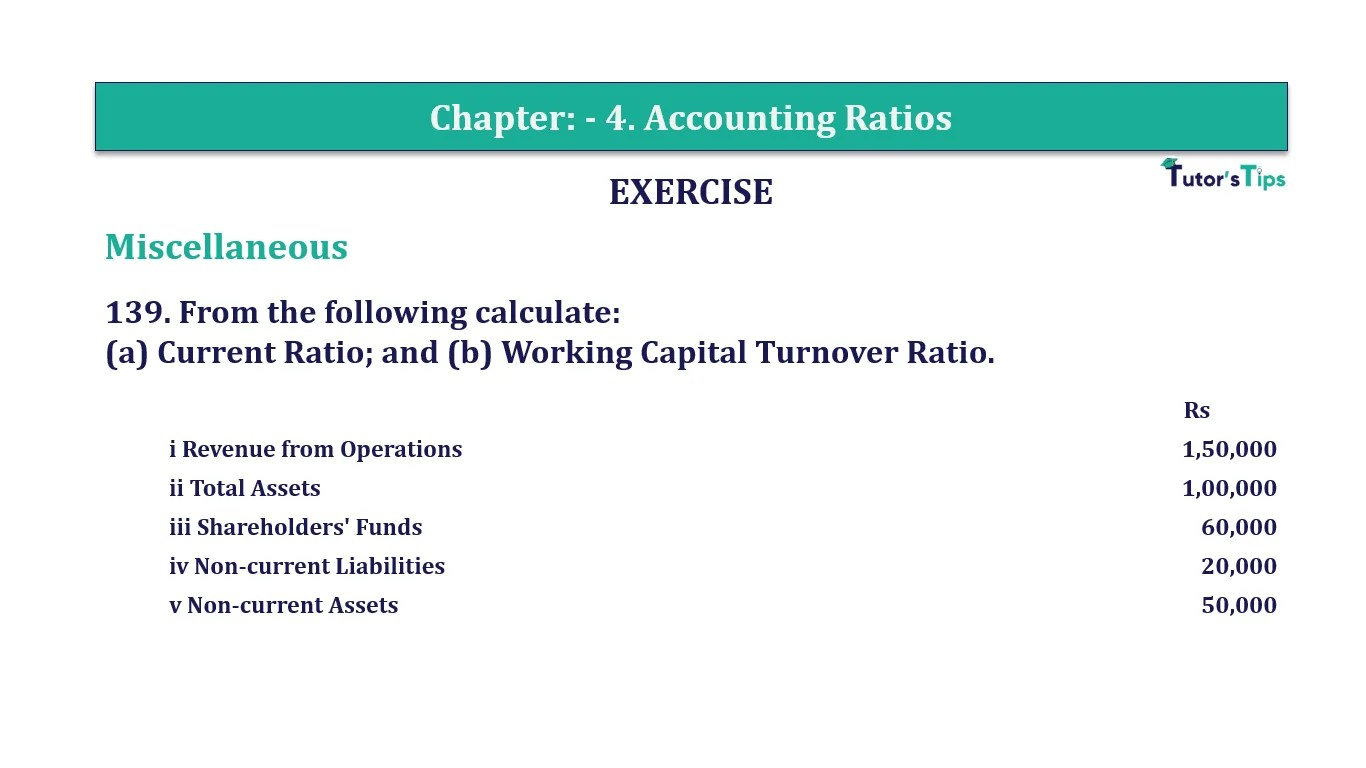Question 139 Chapter 4 of +2-B Miscellaneous 139. From the following calculate:(a) Current Ratio; and (b) Working Capital Turnover Ratio.   Rs i Revenue from Operations 1,50,000 ii Total Assets  1,00,000 iii Shareholders’ Funds  60,000 iv Non-current Liabilities 20,000 v Read More …

## Question 138 Chapter 4 of +2-B – T.S. Grewal 12 Class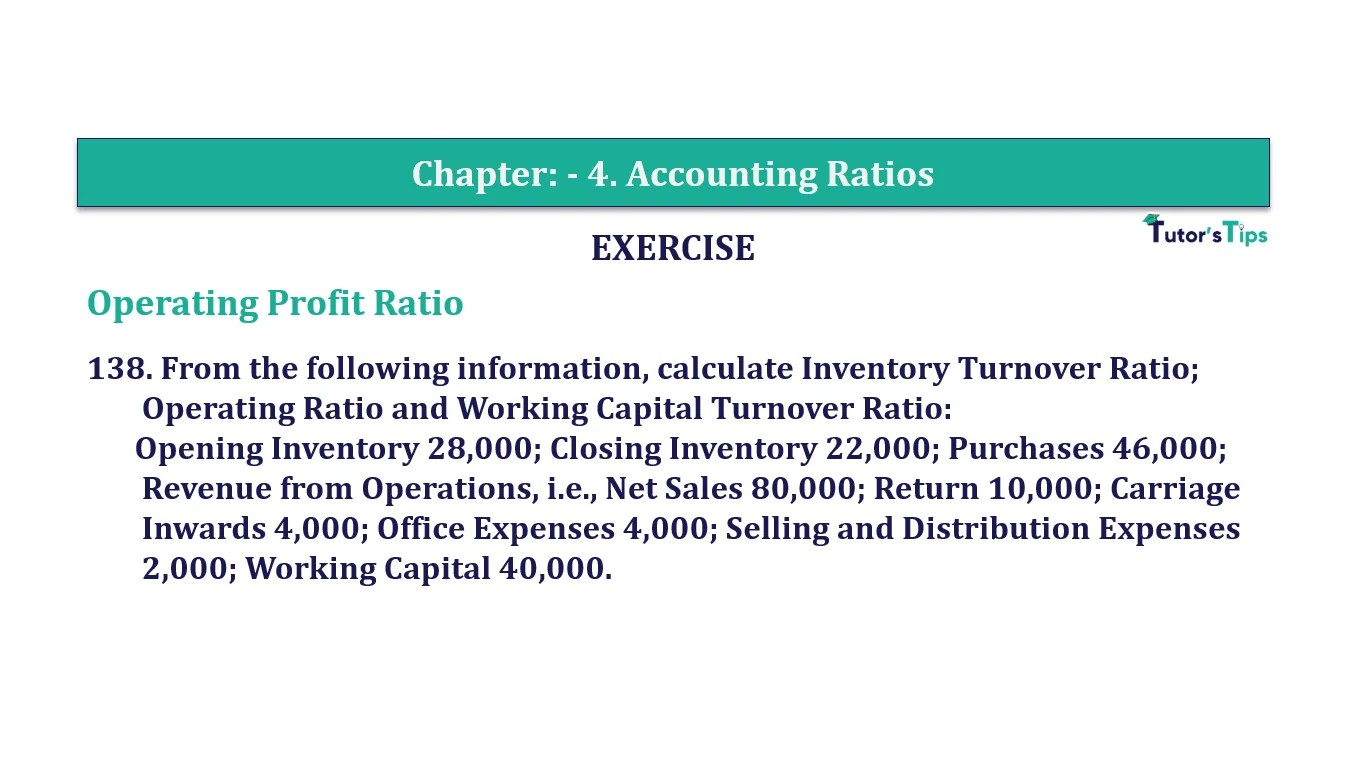Question 138 Chapter 4 of +2-B Miscellaneous 138. From the following information, calculate Inventory Turnover Ratio;Operating Ratio and Working Capital Turnover Ratio:Opening Inventory 28,000; Closing Inventory 22,000; Purchases 46,000;Revenue from Operations, i.e., Net Sales 80,000; Return 10,000; CarriageInwards 4,000; Office Read More …